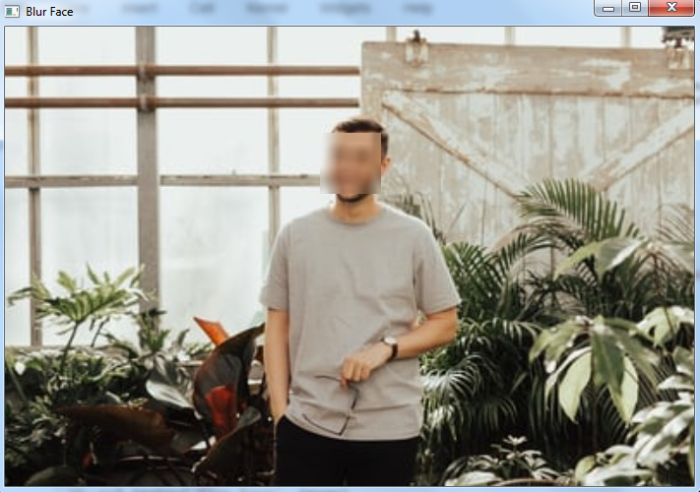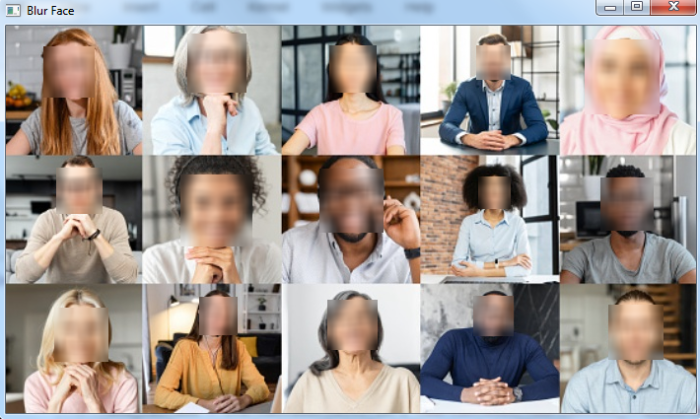# How to blur faces in an image using OpenCV Python?

To blur faces in an image first we detect the faces using a haar cascade classifier. OpenCV provides us with different types of trained haarcascades for object detection. We use haarcascade_frontalface_alt.xml as a haarcascade xml file. To blur the face area, we apply the cv2.GaussianBlur().

## Steps

You could follow the steps given below to blur faces in an image −

• Import the required library. In all the following examples, the required Python library is OpenCV. Make sure you have already installed it.

• Read the input image using cv2.imread(). Specify the full image path.

• Initiate a Haar cascade classifier for face detection as face_cascade = cv2.CascadeClassifier(). Give the full path of the haar cascade xml file. You can use haarcascade_frontalface_alt.xml to detect faces in the image.

• Detect faces in the input image using face_cascade.detectMultiScale(). It returns the coordinates of detected faces in (x,y,w,h) format.

• Define roi as image[y:y+h, x:x+w] for the detected face and apply Gaussian blur to the roi. Add the blurred face to the original image to get the final image.

• Print the image with a blurred face.

Let's have a look at some examples for more clear understanding.

Note: It is very important that you have "haar cascade XML files" in the right folder. Here we put the XML files in a folder named "haarcascades".

## Example

In this example, we blur the face detected in the input image.

# import required libraries
import cv2

# define haar cascade for face detection
print("Face detected in the image:", len(faces))

# Loop over all the detected faces in the image
for (x, y, w, h) in faces:
roi = image[y:y+h, x:x+w]

# apply gaussian blur to face rectangle
roi = cv2.GaussianBlur(roi, (17, 17), 30)

# add blurred face on original image to get final image
image[y:y+roi.shape, x:x+roi.shape] = roi

# Display the output
cv2.imshow('Blur Face', image)
cv2.waitKey(0)
cv2.destroyAllWindows()


## Input Image

Consider the following image as the input image 'man3.jpg' used in the above example.## Output

On execution, it will produce the following output

Face detected in the image: 1


And we get the below window showing the blurred face in the image-## Example

The Python program below demonstrates how to blur faces in an input image.

# import required libraries
import cv2

# define haar cascade for face detection
print("Face detected in the image:", len(faces))

# Loop over all the detected faces in the image
for (x, y, w, h) in faces:
roi = image[y:y+h, x:x+w]

# apply gaussian blur to face rectangle
roi = cv2.GaussianBlur(roi, (15, 15), 30)

# add blurred face on original image to get final image
image[y:y+roi.shape, x:x+roi.shape] = roi

# Display the output
cv2.imshow('Blur Face', image)
cv2.waitKey(0)
cv2.destroyAllWindows()


## Input Image

We will use the following image as the input file for this program −On execution, it will produce the following output:

Face detected in the image: 15


And we get the below window showing the blurred faces in the input imageNotice that all 15 faces are of different sizes. All different faces are blurred.And we get the below window showing the blurred faces in the input imageNotice that all 15 faces are of different sizes. All different faces are blurred.

Note − For better face detection, adjust second and third arguments used in the detectMultiScale() function.